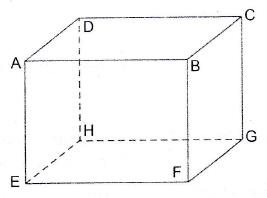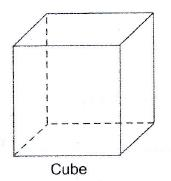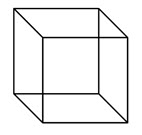Solids: The bodies occupying space are called Solids. Solids could come in all shapes and sizes. Common ones could be cube, cuboid, cones, sphere, etc.

Volume of a solid: The space occupied by a solid body is called its volume. The units of volume are cubic centimeter or$cm^3$ or cubic meters or$m^3$

Cuboid: A solid bounded by six rectangular plane faces it called a cuboid. A cuboid has 6 rectangular faces, 12 edges and 8 vertices.

In the adjoining figure,$ABCDEFGH$ is a cuboid whose1. Six  faces are:$ABCD, EFGH, EFBA, HGCD, EHDA\ and\ BFGC$.
2. Out of these, the four side faces, namely$EFBA, HGCD, EHDA, \ and\ BFGC$ are called lateral faces.
3. 12 edges are:$AB, CD, EF, GH, AE, DH, BF, CG, AD, EH, FG, and BC$.
4. Eight Vertices are:$A, B, C, D, E, F, G, and H$.Cube: A cuboid whose length, breadth and height are all equal is called a cube. Each edge of a cube is called its side.

Formula for Cuboid and Cube

Consider a cuboid with$length=l, \ width=w \ and \ height=h$.$Volume\ of\ a\ cuboid=(l\times b\times h) cubic\ units.$$Total\ surface\ Area\ of\ a\ cuboid=2(lb+bh+lh) \ units.$$Lateral \ surface \ Area \ of \ a \ cuboid = 2(l + b) \times h sq. units.$$Diagonal\ of\ a\ cuboid=\sqrt{l^2+b^2+h^2} \ units$

Consider a cube with all sides equal to$a$ units.$Volume \ of \ a \ cube = a^3 \ cubic \ units.$$Total \ surface \ Area \ of \ a \ cube = 6a^2 \ units$$Lateral \ surface\ Area\ of\ a\ cube=4a^2 \ units.$$Diagonal \ of \ a \ cube = a\sqrt{3}$